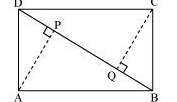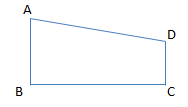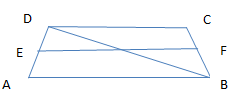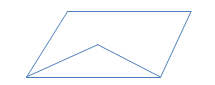# Class 9 Maths Important Questions for Quadrilaterals

Given below are the Class 9 Maths Extra Questions for Quadrilaterals
a. True and False
b. Hard problems
c. Multiple choice questions
e. Fill in the blanks

## True and False

Question 1
Which are these is true or false about parallelogram
1. The diagonals of a parallelogram bisect each other
2. In a parallelogram, opposite sides and angle are equal.
3. A diagonal of a parallelogram divides it into two congruent triangles
4. The bisectors of the angles of parallelogram create a rectangle
5. Sum of all the internal angles is 3600
6. Sum of all the exterior angles is 1800
7. Square, rectangle and rhombus are all parallelogram
8. Consecutive angles are supplementary

Question 2
True or False statement
1. All the angles of the quadrilateral are obtuse
2. Diagonal of the rhombus are equal and perpendicular to each other
3. Diagonal of the square are equal and bisect each other at right angle
4. Out of four points A,B,C,D in place, there are collinear. A quadrilateral can be formed from these points
5. Trapezium, in which the sides that are not parallel are equal in length and angles formed by parallel sides are equal, such trapezium is called isosceles trapezium
6. In a parallelogram, diagonal bisect the angles

## Multiple choice Questions

Question 3
ABCD is a parallelogram and AP and CQ are perpendiculars from vertices A and C on diagonal BDWhich of the following is true based on given information
a. $AP=CQ$
b. $QD=PB$
c. $DP=QB$
d. $\Delta PAD \cong \Delta QCB$

Question 4
The angles of the quadrilateral are in the ratio 2:5:4:1?
Which of the following is true?
a. Largest angle in the quadrilateral is $150^0$
b. Smallest angle is $30^0$
c.The second largest angle in the quadrilateral is $80^0$
d.None of these

Question 5
Two adjacent angles in a parallelogram are in the ratio 2:4. Find the values?
a. 80,100
b. 40,140
c. 60,120
d. None of the above

Question 6
ABCD is a trapezium with AB =10cm, AD=5 cm, BC=4 cm and DC =7 cm?Find the area of the ABCD
a. 34 cm2
b. 28cm2
c. 20 cm2
d. None of these

Question 7
PQRS is a quadrilateral whose diagonal bisect each other at right angles
a. PQRS is a Square
b. PQRS is a rectangle
c. PQRS is a rhombus
d. None of these

Question 8
ABCD is a trapezium where AB||DC. BD is the diagonal and E is the mid point of AD. A line is draw from point E parallel to AB intersecting BC at F. Which of these is true?a. BF=FC
b. EA=FB
c. CF=DE
d. None of these

Question 9
ABCD is a rectangle and P, Q, R and S are mid-points of the sides AB, BC, CD and DA respectively
a. PQRS is a rectangle
b. PQRS is a parallelogram
c. PQRS is a rhombus
d. None of these

Question 10
In a parallelogram PQRS, The bisector of angles P and Q meet at point O as shown in below figure. What is the angle O?a. 80
b. 90
c. 45
d. None of the above

## Match the column

Question 11
 Rhombus Is a quadrilateral with only one pair of parallel sides. Rectangles Is a quadrilateral whose Two pairs of adjacent sides of a kite are equal, and one pair of opposite angles is equal. Diagonals intersect at right angles. One diagonal is bisected by the other. Kite Is a quadrilateral whose all the sides are equal and opposite sides are parallel. Opposite angles are equal. Right-angled trapezoid Is a quadrilateral whose opposite sides of a rectangle are parallel and equal. All angles are 90º. Isosceles trapezoid A trapezoid having two right angles Trapezoid Is a trapezoid whose non parallel sides are equal

Question 12.
Show that the quadrilateral formed by joining the mid- points of adjacent sides of rectangle is a rhombus.

Question 13
P, Q, R and S are respectively the mid- point of sides AB, BC, CD and DA of a quadrilateral ABCD such that AC=BD. Prove that PQRS is a rhombus.

Question 14.
Prove that the quadrilateral formed (if possible) by the internal angular bisectors of any quadrilateral is cyclic.
Question 15.
PQ and RS are to equal and parallel line segments. Any point M not lying on PQ or RS is joined to Q and S and lines through P parallel to QM and through R parallel to SM meet at N. Prove that the line segments MN and PQ are equal and parallel to each other.
Question 16.
l, m and n are three parallel lines intersected by transversal's p and q such that l, m and n cut off equal intercepts AB and BC on p. Show that l, m and n cut off equal intercepts DE and EF on q also.

Question 17.
In a quadrilateral ABCD, CO and DO are bisectors of $\angle C$ and $\angle D$ respectively. Prove that $\angle COD = \frac {1}{2} (\angle A + \angle B)$
Question 18.
ABC is a triangle. D is a point on AB such that AD = ¼ AB and E is the point on A such that AE = ¼ AC. Prove that DE = ¼ BC.
Question 19.
If the diagonals of a parallelogram are equal, then show that it is a rectangle.
Question 20.
In a parallelogram, show that the angle bisectors of two adjacent angles intersect at right angles.
Question 21.
Show that the line segment joining the mid- point of any two sides of a triangle is parallel to third side and is equal to half of it.
Question 22.
A diagonal of a rectangle is inclined to one side of a rectangle at $25^0$. Find the acute angles between the rectangle diagonals.
Question 23.
ABCD is a parallelogram, AD is produced to E so that DE = DC and EC produced meets AB produced in F. Prove that BF = BC.
Question 24.
ABCD is a parallelogram P is a point on AD such that AP = 1/3 AD and Q is a point on BC such that CQ = 1/3 BC. Prove that AQCP is a parallelogram.
Question 25.
P is the mid- point of side AB of a parallelogram ABCD. A line through B parallel to PD meets DC at Q and AD produced at R. Prove that
1. AR = 2BC
2. BR = 2BQ.
Question 26
ABCD is a kite having AB = AD and BC = CD. Prove that the figure formed by joining the mid- points of the side, in order, is a rectangle.

## Summary

This Quadrilaterals class 9 extra questions with answers is prepared keeping in mind the latest syllabus of CBSE . This has been designed in a way to improve the academic performance of the students. If you find mistakes , please do provide the feedback on the mail.